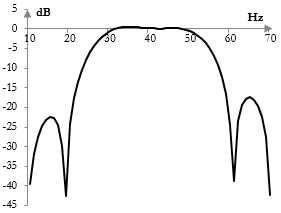# Band pass filter

A band pass filter is a frequency filter that passes the frequencies inside a frequency band and stops the frequencies that are below and above that band.

## Band pass filter example and sound sample

See Orinj Band pass filter to hear a sound sample before and after a band pass filter.

## Example magnitude response of a band pass filter

The following is an example magnitude response of a band pass filter. This filter leaves the magnitudes of frequencies approximately between 25 Hz and 55 Hz unchanged in the signal, but reduces the magnitudes of frequencies below 25 Hz and above 55 Hz.The opposite of a band pass filter is a band stop filter.

A band pass filter with a very narrow pass band is called a peak filter.

## Finite impulse response digital band pass filters

The following is the formula for a finite impulse response (FIR) band pass filter.

$$a(k)=\begin{cases} \frac{ \sin(\frac{2\pi f_1 (k-\frac{N-1}{2}}{f_s})}{\pi (k-\frac{N-1}{2})}-\frac{ \sin(\frac{2\pi f_0 (k-\frac{N-1}{2}}{f_s})}{\pi (k-\frac{N-1}{2})}, & k \ne \frac{N-1}{2}\\ 2\frac{f_1}{f_s}-2\frac{f_0}{f_s}, & k = \frac{N-1}{2}\end{cases}$$

Here, N is the length of the filter. f1 and f0 are the cutoff frequencies at the top and bottom end of the pass band respectively. fs is the sampling frequency.

As the formula shows, the FIR band pass filter is simply the difference between two low pass filters – one at the top cutoff frequency and one at the lower cutoff frequency.

The magnitude response shown in the graph above was created with this formula and with N = 201, f1 = 55 Hz, f0 = 25 Hz, and fs = 2000.

Practical band pass filters are not ideal. They do not completely remove frequencies outside of the pass band. Their magnitude responses may have ripples in the pass and stop bands. The transitions between the pass and stop bands is smooth, rather than sudden. For a discussion of the differences between ideal and actual FIR filters, see Low pass filter.

## Infinite impulse response digital band pass filter

Infinite impulse response (IIR) band pass filters are typically created by substituting (s / ωc) with (s2 + ωc2) / (B s) in the Laplace transform transfer function of an IIR low pass filter. Here, ωc is the mid-point of the pass band and B is the width of the pass band.

An example IIR band pass filter is shown in the topic Butterworth filter.

Other prototype IIR filters include the Bessel filter, Chebychev filter (Type I), Chebychev filter (Type II), and elliptic filter. A band pass filter can also be created with the shelving filter prototype.

### Filtered HTML

• Freelinking helps you easily create HTML links. Links take the form of [[indicator:target|Title]]. By default (no indicator): Click to view a local node.